Related Articles

# How to sort grouped Pandas dataframe by group size ?

• Last Updated : 28 Apr, 2021

In this article, we will discuss how to sort grouped data based on group size in Pandas.

### Functions used

Here we will pass the inputs through the list as a dictionary data structure.

• groupby(): groupby() is used to group the data based on the column values.
• size(): This is used to get the size of the data frame.
• sort_values(): This function sorts a data frame in Ascending or Descending order of passed Column.

The task is straightforward, for a given dataframe first we need to group by any column as per requirement and then arrange the grouped values of the column according to their size. By size here we mean how many times a value has appeared in a column or its frequency.

Example 1:

## Python3

 `# importing pandas module for dataframe``import` `pandas as pd`` ` `# creating a dataframe with student``# name and subject`` ` `dataframe1 ``=` `pd.DataFrame({``'student_name'``: [``'bobby'``, ``'ojaswi'``, ``'gnanesh'``,``                                            ``'rohith'``, ``'karthik'``, ``'sudheer'``,``                                            ``'vani'``],``                            ` `                           ``'subjects'``: [``'dbms'``, ``'python'``, ``'dbms'``, ``'oops'``,``                                        ``'oops'``, ``'oops'``, ``'dbms'``]})`` ` `# display dataframe``print``(dataframe1)`` ` `# group the data  on subjects column based on``# size and sort in descending order``a ``=` `dataframe1.groupby(``'subjects'``).size().sort_values(ascending``=``False``)`` ` `# group the data  on subjects column based on ``# size and sort in ascending order``b ``=` `dataframe1.groupby(``'subjects'``).size().sort_values(ascending``=``True``)`` ` `print``(a, b)`

Output: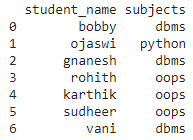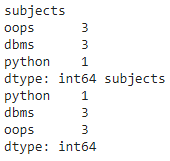Example 2:

## Python3

 `# importing pandas module for dataframe``import` `pandas as pd`` ` `# creating a dataframe with student name``# , subject and address``dataframe1 ``=` `pd.DataFrame({``'student_name'``: [``'bobby'``, ``'ojaswi'``, ``'gnanesh'``,``                                            ``'rohith'``, ``'karthik'``, ``'sudheer'``,``                                            ``'vani'``],``                           ``'subjects'``: [``'dbms'``, ``'python'``, ``'dbms'``, ``'oops'``, ``                                        ``'oops'``, ``'oops'``, ``'dbms'``],``                            ` `                           ``'address'``: [``'ponnur'``, ``'ponnur'``, ``'hyd'``, ``'tenali'``,``                                       ``'tenali'``, ``'hyd'``, ``'patna'``]})`` ` `# display dataframe``print``(dataframe1)`` ` `# group the data  on address column based  ``# on size and sort in descending order``a ``=` `dataframe1.groupby(``'address'``).size().sort_values(ascending``=``False``)`` ` `# group the data  on address column based ``# on size and sort in ascending order``b ``=` `dataframe1.groupby(``'address'``).size().sort_values(ascending``=``True``)`` ` `print``(a, b)`

Output: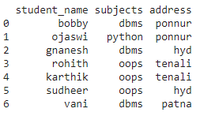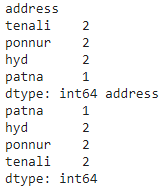We can also group the multiple columns. The syntax remains the same, but we need to pass the multiple columns in a list and pass the list in groupby()

Syntax:

dataframe.groupby([column1,column2,.column n]).size().sort_values(ascending=True)

Example 3:

## Python3

 `# importing pandas module for dataframe``import` `pandas as pd`` ` `# creating a dataframe with student``# name , subject and address``dataframe1 ``=` `pd.DataFrame({``'student_name'``: [``'bobby'``, ``'ojaswi'``, ``'gnanesh'``,``                                            ``'rohith'``, ``'karthik'``, ``'sudheer'``,``                                            ``'vani'``],``                            ` `                           ``'subjects'``: [``'dbms'``, ``'python'``, ``'dbms'``, ``'oops'``,``                                        ``'oops'``, ``'oops'``, ``'dbms'``],``                            ` `                           ``'address'``: [``'ponnur'``, ``'ponnur'``, ``'hyd'``, ``'tenali'``,``                                       ``'tenali'``, ``'hyd'``, ``'patna'``]})`` ` `# display dataframe``print``(dataframe1)`` ` `# group the data  on address and subjects``# column based on size and sort in descending``# order``a ``=` `dataframe1.groupby([``'address'``, ``'subjects'``]``                       ``).size().sort_values(ascending``=``False``)`` ` `# group the data  on address and subjects``# column based on size and sort in ascending``# order``b ``=` `dataframe1.groupby([``'address'``, ``'subjects'``]``                       ``).size().sort_values(ascending``=``True``)`` ` `print``(a, b)`

Output: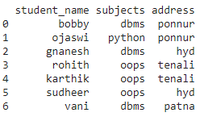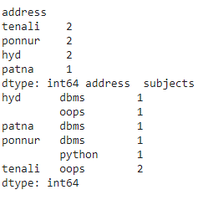Attention geek! Strengthen your foundations with the Python Programming Foundation Course and learn the basics.

To begin with, your interview preparations Enhance your Data Structures concepts with the Python DS Course. And to begin with your Machine Learning Journey, join the Machine Learning – Basic Level Course

My Personal Notes arrow_drop_up